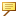Descriptive Analytics

Descriptive Analytics
Descriptive Analytics provides on-demand services that perform common statistical calculations and facilitate statistical monitoring. When using the ThingWorx platform to build an IoT solution, output from these services can be added quickly and easily to an application. The services provided by Descriptive Analytics can be used to build solution-specific visualizations (mashups or widgets), create alerts based on logged property values, or generate transformed data for machine learning processes.
The Descriptive Analytics microservice includes two microservers, each with its own set of statistical services.
StatisticalCalculation Microserver
The StatisticalCalculation Microserver contains a library of computational services that perform common statistical calculations and frequency transformations on raw data. These services are designed to receive data from the QueryTimedValuesForProperty service.Use of the QueryTimedValuesForProperty service is not a requirement. However, when data is provided from other sources, the format of the data might cause error during execution.
The StatisticalCalculation Microserver include the following services.
Calculation Service*
Description
Binned Data Distribution for Bin Size
Calculate the binned distribution of data points based on the desired bin size.
Binned Data Distribution for Number of Bins
Calculate the binned distribution of data points based on the desired number of bins.
Confidence Interval Values
Based on a specified confidence interval percentage, calculate the minimum, median, and maximum interval values.
Five Number Property Values
Calculate the five number values: minimum, lower quartile, median, upper quartile, and maximum.
Fourier Transform
Calculate the results of running the fast Fourier transform on the specified values.
Maximum Value
Calculate the maximum property value in the provided infotable.
Mean Value
Calculate the mean property value in the provided infotable.
Median Value
Calculate the median property value in the provided infotable.
Minimum Value
Calculate the minimum property value in the provided infotable.
Mode Value
Calculate the mode values from the provided infotable.
Sampling Frequency Values
Calculate the sampling frequency values: minimum, median, and maximum.
Standard Deviation Value
Calculate the standard deviation value from the provided infotable.
Range Value
Calculate the range of property values in the provided infotable, from the maximum to the minimum.Available only from release 8.4.4 onward.
Rate of Change
Calculate the percentage of difference between two specified values.
* Most of the Statistical Calculation services do not use the timestamp that is included in the data. Only the Fourier Transform and the Sampling Frequency Values services use the timestamp. For these services the timestamp parameter must be formatted according to the output of the QueryTimedValuesForProperty service.
StatisticalMonitoring Microserver
The StatisticalMonitoring Microserver contains a library of services that calculate the following types of statistical control criteria on a stream of data:
 Monitoring Service Description Number of Points Beyond a Threshold Monitor for the number of points in a series that are above or below a defined threshold. Number of Consecutive Points Beyond a Threshold Monitor for the number of consecutive point in a series that are above or below a defined threshold. Number of Points Based on a Range Monitor for the number of points in a series that remain inside or outside of a defined range. Number of Consecutive Points Based on a Range Monitor for the number of consecutive points in a series that remain inside or outside of a defined range. Number of Consecutive Points Following a Trend Monitor for the number of consecutive points in a series that are increasing, decreasing, or alternating direction. Rate of Change Monitor for the percentage of difference between two specified values. Number of Points with Change Rate Out of Range Monitor for how many pairs of consecutive points in a series have a rate of change outside the defined range. If the first value in a pair is 0, the pair is not considered for rate of change.
Related Topics